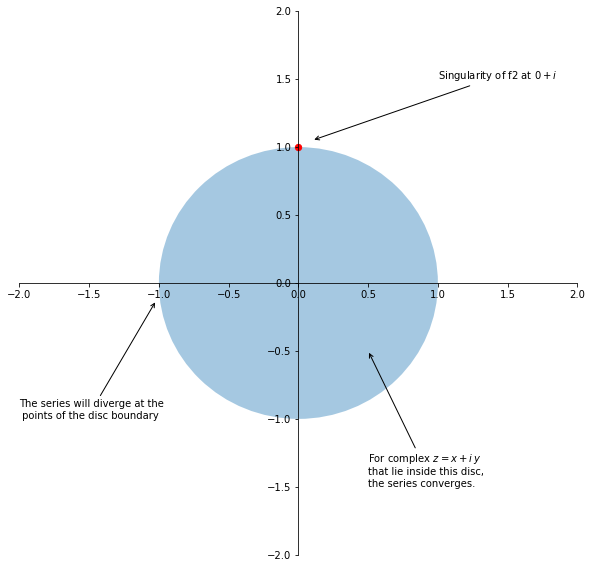## Taylor expansions¶

We first import some prerequisite modules, most importantly Sympy and Matplotlib.

In :
def uniq(seq):
# order preserving
seen = {}
result = []
for item in seq:
if item in seen:
continue
seen[item] = 1
result.append(item)
return result

In :
import sympy as sm
from sympy.abc import x
from matplotlib.colors import cnames

In :
import matplotlib
from matplotlib.colors import rgb2hex

from numpy import arange

In :
from sympy import Symbol,plot
import matplotlib.pyplot as plt

def move_sympyplot_to_axes(p, ax):
backend = p.backend(p)
backend.ax = ax
backend._process_series(backend.parent._series, ax, backend.parent)
#     Before Sympy 1.5, replace the above line with backend.process_series()
backend.ax.spines['right'].set_color('none')
backend.ax.spines['bottom'].set_position('zero')
backend.ax.spines['top'].set_color('none')
plt.close(backend.fig)


The following routine will plot the function f together with its Taylor polynomials up to the degree degree, in the square box with sides [-A,A]. We will use it several times for different functions f.

In :
def taylorplot(f, degree, A):
L = [f.series(n=i).removeO() for i in range (1, degree+2)] # +1 for the offset of range, another +1 for the offset of .series
L = uniq(L)
L.insert(0,f)
# Pick colors from the Blues colorscheme
a = arange(0,1,1/len(L))
c = matplotlib.cm.Blues(a)[:,:3]

# Plot the original function in red, then add the graphs of Taylor polynomials in different shades of blue
p1 = sm.plot(L, xlim=[-A,A], ylim=[-A,A], line_color = 'r',  show=False)
for i in range(1,len(L)):
p1.extend(sm.plot(L[i], xlim=[-A,A], ylim=[-A,A], line_color = rgb2hex(c[i,:]), show=False))
p1.legend = False
# p1.show()

# Plot the original function alone
p2 = sm.plot(L, xlim=[-A,A], ylim=[-A,A], line_color = 'r', show=False)
p2.legend = False
p2.grid = True
# p2.show()

# Generate the legend by LaTeXing the expressions for the functions involved.
l =[]
for i in range(len(L)):
l.append(sm.latex(L[i], mode='inline'))

# %matplotlib gtk3 # uncomment to use the external plot window
# Combine the two resulting plots, add legend
fig, (ax1,ax2) = plt.subplots(ncols=2,figsize=(20,10))
move_sympyplot_to_axes(p1, ax1)
move_sympyplot_to_axes(p2, ax2)
fig.legend(l,loc='lower center', bbox_to_anchor=(0.5, 0.0),
plt.show()


Let us first plot the partial sums of the Maclaurin series of the Bessel function $J_0$, up to the degree 14. We will restrict the graphs to the box [-10,10] by [-10,10]:

In :
B = sm.besselj(0,x)
taylorplot(B, 14, 10)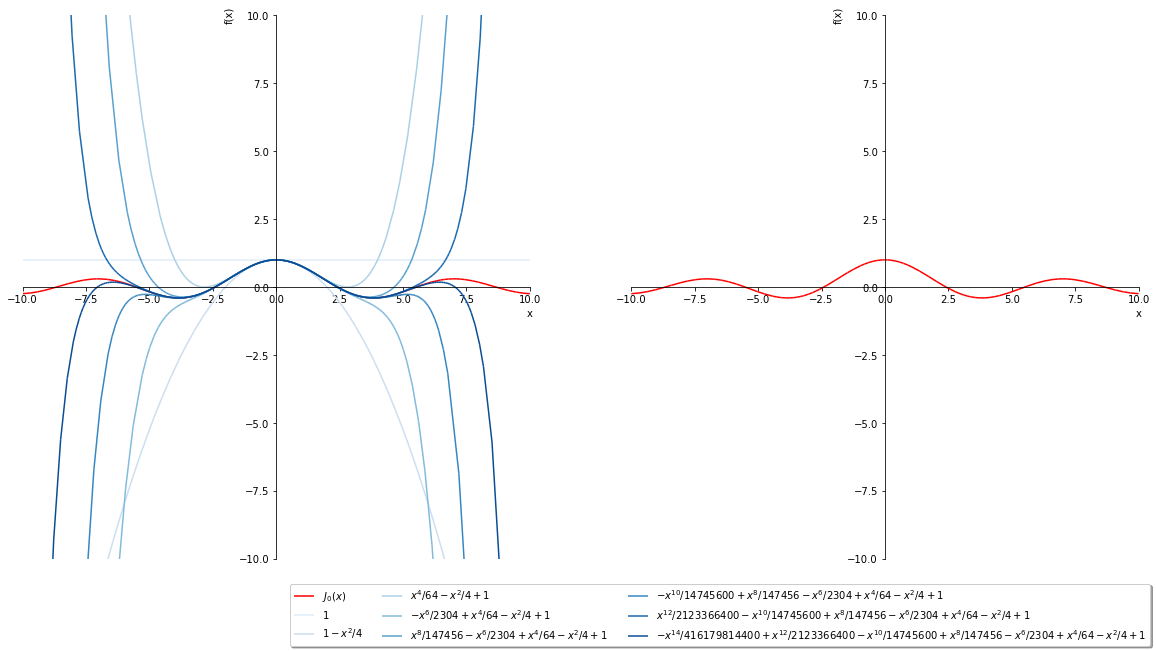In these graphs we show the Taylor polynomials (centered at $a=0$ here), using the darker shade to denote to the higher degree polynomial $T_n(x)$. The plot on the right shows $J_0(x)$ itself.

Next, let us do the same with $\sin(x)$. We plot its Taylor polynomials at 0 up to degree 13 in the box [-10,10] by [-10,10].

In :
S = sm.sin(x)
taylorplot(S, 13,10)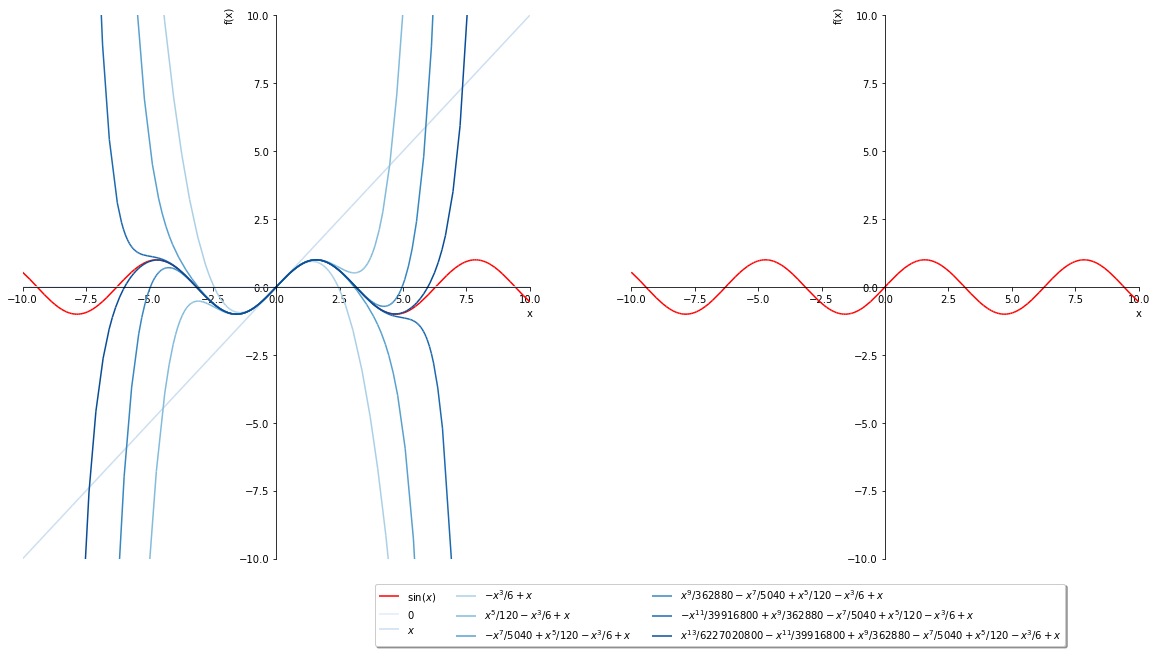Finally, let us look at two examples in which the Maclaurin series of a function does not converge for all x. The first example is $1/(1-x)$; we will plot its Taylor polynomials up to degree 10 in the square box [-6,6] by [-6,6].

In :
f1 = 1/(1-x)
taylorplot(f1,10,6)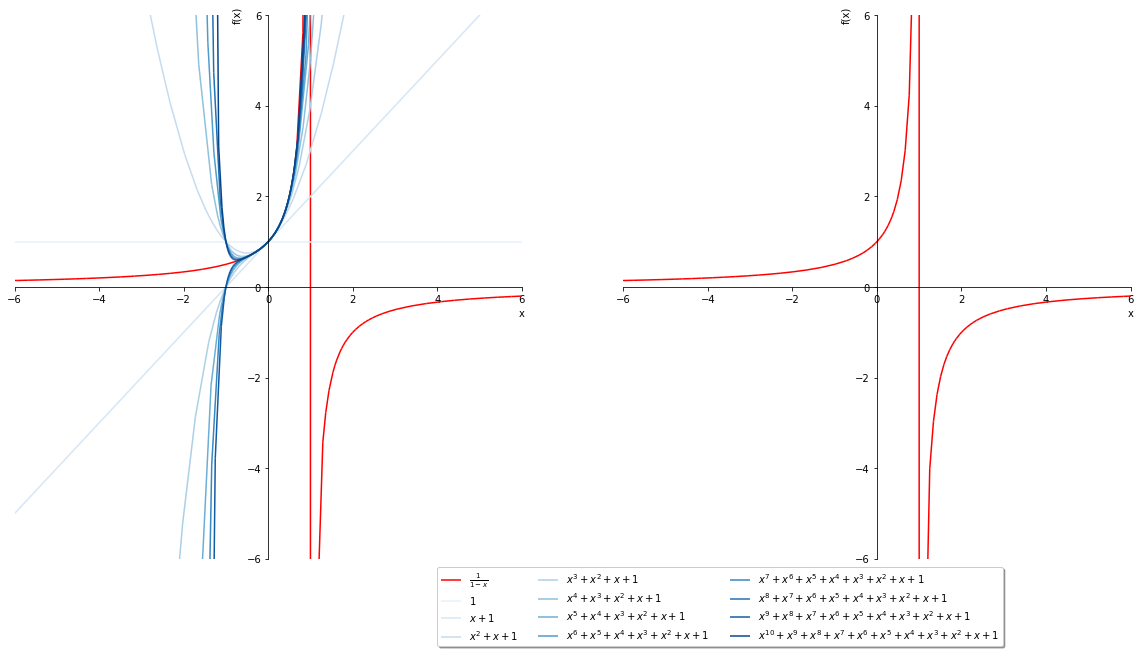Observe how the polynomials $T_n$ diverge away from the graph of the function f1 when $x=-1$, even though the function does not have a singularity there. This is of course because the radius of convergence of the geometric series $$\sum_{n=0}^\infty x^n$$ is $1$. Since the interval of convergence of a series must be symmetric, divergence of the series at $x = 1$, where the function f1 has a singularity, means that the series must also diverge at $x = -1$.

The following example is even more subtle. The function we look at here, f2$= 1/(1+x^2)$, does not exhibit any singularities at all! On the other hand, its Maclaurin series $$\sum_{n=0}^\infty\, (-1)^n x^{2n}$$ diverges as soon as $|x|\geq 1$. Let us plot the Taylor polynomials at $a = 0$ up to the degree $n = 20$.

In :
f2 = 1/(1+x**2)
taylorplot(f2,20,6)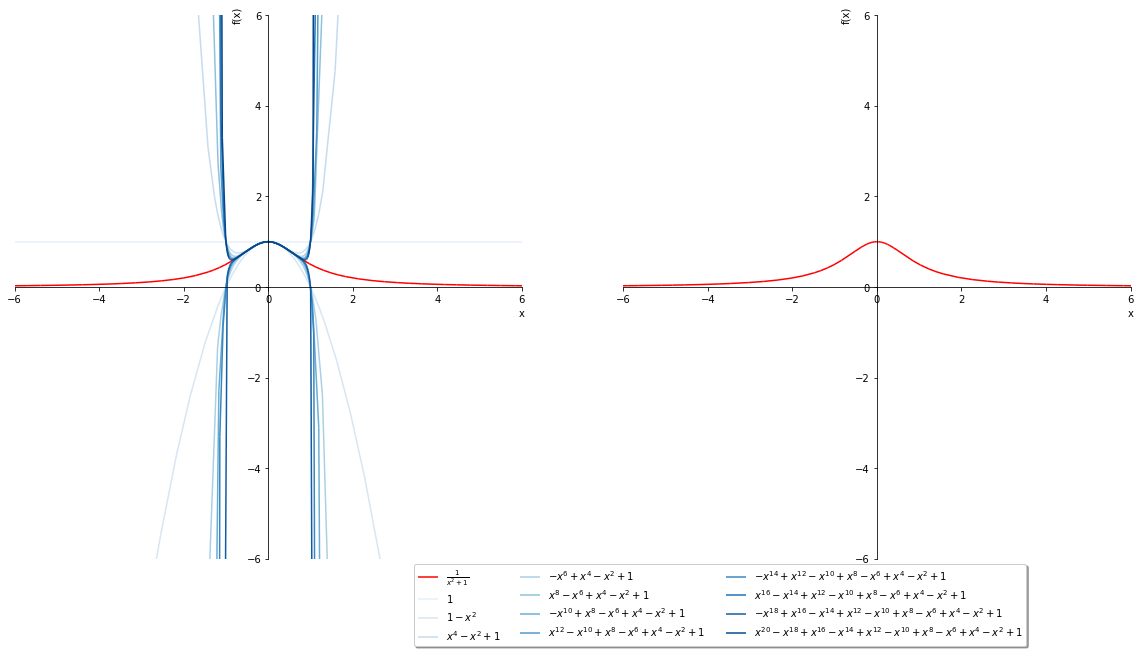Outside of Calc2: It turns out, to understand the reason for such behavior of this series, one has to extend the function f2$=1/(1+x^2)$ to complex numbers, where for $x = i$, our function f2 has a singularity (the complex number $i$ is such that $i^2+1 = 0$, turning the denominator of f2 into $0$). In the complex plane, series converge on discs instead of intervals, so because the function f2 has a singularity at the point $0+i\,1$, its series centered at $a=0$ will have the radius of convergence equal to $1$. We show this relation in the following graph. In it, a point $(x,y)$ in the plane stands for the complex number $z = x+ iy$.

In :
    fig, ax = plt.subplots(figsize=(10,10))
circle =  matplotlib.patches.Circle ((0, 0), 1, edgecolor='none',alpha=0.5)
p = matplotlib.collections.PatchCollection([circle], alpha=0.4)
ax.scatter([0.0],[1.0],marker='o',c='r',s=40)
ax.annotate('Singularity of f2 at $0+i$', xy=(0.1, 1.05), xytext=(1, 1.5),
arrowprops=dict(arrowstyle="->")
)
ax.annotate('For complex $z= x + i\,y$\nthat lie inside this disc,\nthe series converges.', xy=(0.5, -0.5), xytext=(0.5, -1.5),
arrowprops=dict(arrowstyle="->")
)
ax.annotate('The series will diverge at the\n points of the disc boundary', xy=(-1.02, -0.13), xytext=(-2, -1),
arrowprops=dict(arrowstyle="->")
)
# This adjusts the position of the axes
ax.spines['left'].set_position('center')
ax.spines['bottom'].set_position('center')
ax.spines['right'].set_color('none')
ax.spines['top'].set_color('none')
ax.xaxis.set_ticks_position('bottom')
ax.yaxis.set_ticks_position('left')

plt.ylim(-2,2)
plt.xlim(-2,2)
plt.show()